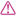Below is a list of all the Keywords, Operators, and Literals that area reserved phrases in FiM++. These are in alphabetical order, sorted by purpose.

## Keywords

Conditional conclusion END_IF

• `That's what I would do`

Conditional conclusion END_ELSE

• `That's what I would do`

Conditional conclusion END_SWITCH

• `That's what I did`

Conditional DEFAULT

• `If all else fails`

Conditional prefix ELSE

• `Or else`
• `Otherwise`

Conditional prefix CASE

• `On the`
• partner suffix
• `hoof`
• `nd hoof`
• `rd hoof`
• `st hoof`
• `th hoof`

Conditional prefix IF

• `If`
• `When`
• partner suffix
• `then`

Conditional prefix SWITCH

• `In regards to`

Declaration of reports

• `Dear`

Declaration of paragraphs

• `I learned`
• Declaration of mane paragraphs
• `Today I learned`

Definition of variable

• `Did you know that`

Define the type of variable returned by a paragraph

• `to get`
• `with`

End of report

• `Your faithful student`

End of paragraph

• `That’s all about`

Import a report

• `Remember when I wrote about`

Initialization of variables

• `has`
• `is`
• `like`
• `likes`
• `was`

Initialization of constants

• `always has`
• `always is`
• `always like`
• `always likes`
• `always was`

Listing imported interfaces

• `and`

Listing paragraph parameters

• `using`

Looping conclusion END_DO_WHILE

• `I did this while`
• `I did this as long as`

Looping conclusion END_WHILE

• `That's what I did`

Looping prefix DO_WHILE

• `Here's what I did`

Looping prefix WHILE

• `As long as`
• `while`

Print the following line

• `I said`
• `I sang`
• `I wrote`

Prompt the user for input

• `I asked`

Read a line from the default input stream (typically, the user)

• `I heard`
• `I read`

Return the following value from the current paragraph

• `Then you get`

Run the following paragraph

• `I remembered`
• `I would`

Variable type for a single Boolean value

• `an argument`
• `argument`
• `the argument`
• `logic`
• `the logic`

Variable type for an array of Boolean values

• `arguments`
• `the arguments`
• `logics`
• `the logics`

Variable type for a single 64-bit number

• `a number`
• `number`
• `the number`

Variable type for a single UTF-8 character

• `a character`
• `a letter`
• `character`
• `letter`
• `the character`
• `the letter`

Variable type for an array of 64-bit numbers

• `many numbers`
• `numbers`
• `the numbers`

Variable type for an array of arrays of UTF-8 characters

• `many phrases`
• `many quotes`
• `many sentences`
• `many words`
• `phrases`
• `quotes`
• `sentences`
• `the phrases`
• `the quotes`
• `the sentences`
• `the words`
• `words`

Variable type for an array of UTF-8 characters

• `a phrase`
• `a quote`
• `a sentence`
• `a word`
• `characters`
• `letters`
• `phrase`
• `quote`
• `sentence`
• `the characters`
• `the letters`
• `the phrase`
• `the quote`
• `the sentence`
• `the word`
• `word`

Variable value assignment

• `are now`
• `become`
• `becomes`
• `is now`
• `now like`
• `now likes`

## Operators

• `added to`
• `and`
• `plus`

Arithmetic infix operator (DIVISION)

• `divided by`

Arithmetic infix operator (MULTIPLICATION)

• `multiplied with`
• `times`

Arithmetic infix operator (SUBTRACTION)

• `minus`
• `without`

Arithmetic postfix operator decrement

• `got one less`

Arithmetic postfix operator increment

• `got one more`

• `add`
• `and`

Arithmetic prefix operator (DIVISION)

• `divide`
• `and`
• `by`

Arithmetic prefix operator (MULTIPLICATION)

• `multiply`
• `the product of`
• `and`
• `by`

Arithmetic prefix operator (SUBTRACTION)

• `subtract`
• `the difference between`
• `and`
• `from`

Arithmetic prefix operator decrement

• `There was one less`
• `subtract`
• `from`

Arithmetic prefix operator increment

• `There was one more`
• `add`
• `to`

Boolean logical infix (AND)

• `and`

Boolean logical infix (OR)

• `or`

Boolean logical prefix (EXCLUSIVE OR)

• `either`
• `or`

Boolean logical prefix (NOT)

• `not`
• `it's not the case that`

Compare two values for equality (infix EQUAL)

• `had`
• `has`
• `is`
• `was`
• `were`

Compare two values for inequality (infix NOT EQUAL)

• `hadn't`
• `had not`
• `hasn't`
• `has not`
• `isn't`
• `is not`
• `wasn't`
• `was not`
• `weren't`
• `were not`

Compare two values for inequality (infix GREATER THAN)

• `had more than`
• `has more than`
• `is greater than`
• `is more than`
• `was greater than`
• `was more than`
• `were greater than`
• `were more than`

Compare two values for inequality (infix GREATER THAN OR EQUAL)

• `had no less than`
• `has no less than`
• `is no less than`
• `is not less than`
• `isn't less than`
• `was no less than`
• `was not less than`
• `wasn't less than`
• `were no less than`
• `were not less than`
• `weren't less than`

Compare two values for inequality (infix LESS THAN)

• `had less than`
• `has less than`
• `is less than`
• `was less than`
• `were less than`

Compare two values for inequality (infix LESS THAN OR EQUAL)

• `had no more than`
• `has no more than`
• `is no greater than`
• `is no more than`
• `is not greater than`
• `is not more than`
• `isn't greater than`
• `isn't more than`
• `was no greater than`
• `was no more than`
• `was not greater than`
• `was not more than`
• `wasn't greater than`
• `wasn't more than`
• `were no greater than`
• `were no more than`
• `were not greater than`
• `were not more than`
• `weren't greater than`
• `weren't more than`

## Literals

Boolean literal TRUE

• `correct`
• `right`
• `true`
• `yes`

Boolean literal FALSE

• `false`
• `incorrect`
• `no`
• `wrong`

Non-value literal NULL

• `nothing`